# Introduction

Comparing samples defined over a single dimension is a straightforward task that relies on standard, well established methods. Meanwhile distance between samples in high dimensional space remains a largely unexplored field. Available solutions rely on multivariate normal distributions, a condition that is both difficult to check and overlooking key behaviors in biological samples, where populations of interest often correspond to a small proportion (<1%) of all the points that have been measured.

We have developed hilbertSimilarity to address the problem of sample similarity in mass cytometry where samples are measured over up to 100 dimensions, and where each sample contains a slightly different number of points (or cells). Our method first transforms each sample into a probability vector, by dividing each dimension into a fixed number of bins and by associating each cell to a specific multidimensional cube. The proportion of cells in each hypercube is characteristic of a given sample. To characterize an compare samples we use measures from Information Theory, since their interpretation in terms of similarity and complexity is straightforward.

To demonstrate the power of hilbertSimilarity we applied the method to a subset of the bodenmiller et al. dataset, comparing the effect of different stimulations and identifying groups of cells that are significantly affected by different treatments.

Compared to other methods, hilbertSimilarity does not rely on expert-driven gating, or require any hypothesis about the number of populations that are present in the data. This makes it a powerful tool to quickly assess a dataset before using traditional methods, or when populations a not known a priori.

# Installation

hilbertSimilarity can be installed using the following command

devtools::install_github(yannabraham/hilbertSimilarity)

Once installed the package can be loaded using the standard library command.

library(hilbertSimilarity)

We first need data from the bodenmiller package:

library(bodenmiller)
data(refPhenoMat)
data(untreatedPhenoMat)
data(refFuncMat)
data(untreatedFuncMat)
data(refAnnots)
refAnnots$Treatment <- 'reference' data(untreatedAnnots) fullAnnots <- rbind(refAnnots[,names(untreatedAnnots)], untreatedAnnots) fullAnnots$Treatment <- factor(fullAnnots$Treatment) fullAnnots$Treatment <- relevel(fullAnnots$Treatment,'reference') refMat <- cbind(refPhenoMat,refFuncMat) untreatedMat <- cbind(untreatedPhenoMat, untreatedFuncMat) fullMat <- rbind(refMat,untreatedMat) fullMat <- apply(fullMat,2,function(x) { qx <- quantile(x,c(0.005,0.995)) x[x<qx] <- qx x[x>qx] <- qx return(x) }) In this dataset 51936 cells corresponding to 4 have been measured over 23 channels. Cells have been gated into 14 populations. The percentage of each population per treatment is shown below: ## ## Attaching package: 'dplyr' ## The following objects are masked from 'package:stats': ## ## filter, lag ## The following objects are masked from 'package:base': ## ## intersect, setdiff, setequal, union ## # A tibble: 14 x 5 ## Cells reference BCR/FcR-XL PMA/Ionomycin vanadate ## <fct> <dbl> <dbl> <dbl> <dbl> ## 1 cd14-hladr- 0.14 0.12 0.17 0.43 ## 2 cd14-hladrhigh 0.38 0.12 0.03 0.02 ## 3 cd14-hladrmid 1.55 0.98 0.37 0.28 ## 4 cd14-surf- 11.4 12.1 10.4 28.9 ## 5 cd14+hladr- 0.08 0.11 0.1 1.44 ## 6 cd14+hladrhigh 0.13 0.02 0.03 0.02 ## 7 cd14+hladrmid 8.69 5.5 2.04 2.45 ## 8 cd14+surf- 0.51 0.5 1.47 2.63 ## 9 cd4+ 25.3 26.7 31.2 7.96 ## 10 cd8+ 32.1 33.4 35.1 19 ## 11 dendritic 0.37 0.2 0.19 0.36 ## 12 igm- 1.47 2.16 1.24 1.42 ## 13 igm+ 6.77 5.66 6.81 6.25 ## 14 nk 11.1 12.4 10.8 28.8 The smallest cell population, cd14+hladrhigh, corresponds to 0.058% of the total cells. To demonstrate the use of hilbertSimilarity we will compare analyze the data at the treatment level. # Defining a grid to compare samples The make.cut function is used to prepare the grid that will be used to process the data matrix. It requires 2 arguments: the number of cuts (or bins), and the minimum number of cells to consider for a density. The latter depends on the technology and on the decision by the scientist running the analysis. For CyTOF we will require a population to correspond to at least 40 cells. ## Choosing a number of bins The number of bins in each dimension is defined as follows: $c^{d} < N$ Where $$c$$ is the number of bins, $$d$$ is the number of dimensions and $$N$$ is the total number of cells in the dataset. We chose the first integer $$c$$ that would generate at least 1 bin per cell. Because many combinations don’t have any biological sense, the fraction of occupied bins will be lower than 1. $$c$$ can be computed easily using the following formula $c = \max \left \{ \left \lfloor \sqrt[d]{N} \right \rfloor , 2 \right \}$ The formula has been implemented in the hilbert.order function. For our example, where $$N$$ is 51936 and $$d$$ is 23, $$c$$ is 2. The number of cuts to generate is the number of required bins plus 1. After manual inspection, we used a $$c$$ of 3 to compute the cuts: nbins <- 2 cuts <- make.cut(fullMat, n=nbins+1, count.lim=40) ## CD20 ## IgM ## CD4 ## CD33 ## HLA.DR ## CD14 ## CD7 ## CD3 ## CD123 ## pStat1 ## pSlp76 ## pBtk ## pPlcg2 ## pErk ## pLat ## pS6 ## pNFkB ## pp38 ## pStat5 ## pAkt ## pSHP2 ## pZap70 ## pStat3 ## Reviewing the grid The make.cut function returns 2 cuts: • fixed corresponds to equally sized bins over the range of the channel • combined adjusts the bin size to the density of the channel The cuts can be visualized using the show.cut function: show.cut(cuts)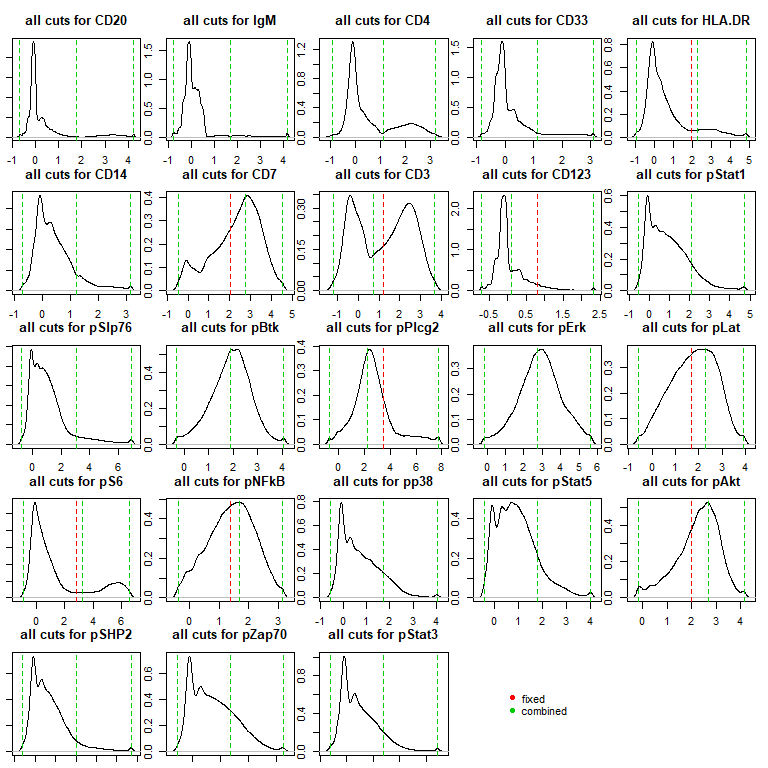The green lines correspond to the fixed limits, the red lines correspond to the adjusted limits (when applicable). For cases like CD3, pSlp76 and ZAP70, it allows for a better separation between the positive and negative populations. # Applying the grid to the data Given a dataset and a grid, the do.cut function will assign each cell to a particular bin in every dimension. cutFullMat <- do.cut(fullMat,cuts,type='combined') Effectively, each cell is now associated to a voxel, and each voxel is enriched for a particular cell type that corresponds to the the unique combination of dimensions and ranges it corresponds to. To uniquely identify each occupied voxel, one can compute a Hilbert index over the grid. The after the dimensions have been ordered to better capture the ## Ordering the dimensions Intuitively, each voxel on the grid contains a specific cell type. In order for populations to be associated with consecutive specific bins, one must optimize the order of channels so that dimensions that are specific to a population are grouped together in the input matrix. A simple way to achieve this is to use the normalized mutual information between the dimensions to cluster them into meaningful groups: library(entropy) miFullMat <- matrix(0,nrow=ncol(fullMat),ncol = ncol(fullMat) ) for (i in seq(ncol(fullMat)-1)) { for (j in seq(i+1,ncol(fullMat))) { cur.tbl <- table(cutFullMat[,i],cutFullMat[,j]) nent <- 1-mi.empirical(cur.tbl)/entropy.empirical(cur.tbl) miFullMat[i,j] <- miFullMat[j,i] <- nent } } dimnames(miFullMat) <- list(colnames(fullMat),colnames(fullMat)) hcFullMat <- hclust(as.dist(miFullMat)) plot(hcFullMat)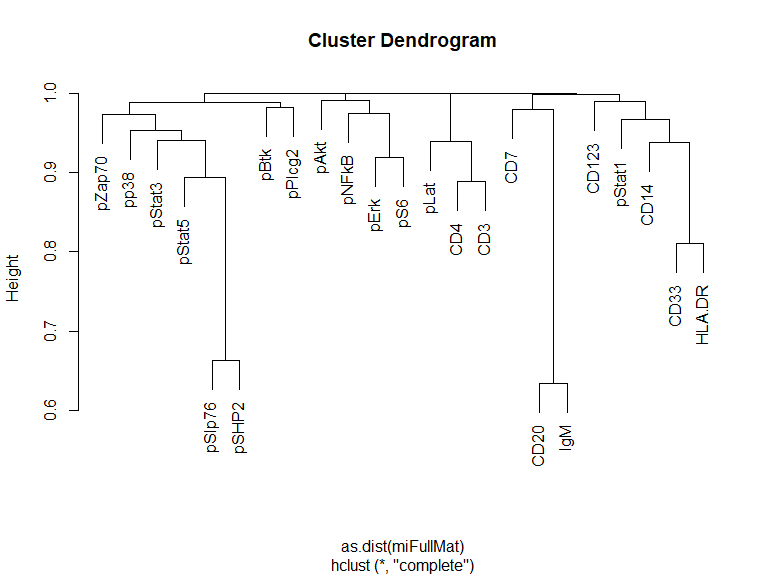## Calculating the Hilbert index After ordering the cut matrix using the normalized mutual information, it can be transformed into a Hilbert index using the do.hilbert function: col.order <- hcFullMat$labels[rev(hcFullMat$order)] hc <- do.hilbert(cutFullMat[,col.order],nbins) All cells are found in 13484 voxels out of the 8.38860810^{6} possible voxels defined over the initial 23-dimensional space (0.16%). # Visualizing the Hilbert curve ## Using the Andrews curve to visualize cell densities Using a Hilbert index to describe the grid has the advantage that consecutive index correspond to consecutive voxels in the grid. Effectively it corresponds to a projection from N dimensions to a single one. To visualize the Hilbert curve we use Andrews plots to standardize the display to a common range. First we compute the number of cells per Hilbert index, per treatment: treatment.table <- with(fullAnnots, table(hc,Treatment)) treatment.pc <- apply(treatment.table,2,function(x) x/sum(x)) Next we prepare the Andrews vector; adjust the number of breaks to return a smoother curve : av <- andrewsProjection(t(treatment.pc),breaks=30) Then we project the Hilbert curve of each treatment onto the Andrews vector: treatment.proj <- t(treatment.pc) %*% t(av$freq)

Now we can visualize the different treatment using line charts:

library(ggplot2)
library(reshape2)
##
## Attaching package: 'reshape2'
## The following object is masked from 'package:tidyr':
##
##     smiths
melt(treatment.proj) %>%
rename(AndrewsIndex=Var2) %>%
mutate(AndrewsIndex=av$i[AndrewsIndex]) %>% ggplot(aes(x=AndrewsIndex,y=value))+ geom_line(aes(group=Treatment,color=Treatment))+ theme_light(base_size=16)+ theme(axis.title.x=element_blank(), axis.text.x=element_blank(), axis.ticks.x=element_blank(), axis.title.y=element_blank(), axis.text.y=element_blank(), axis.ticks.y=element_blank())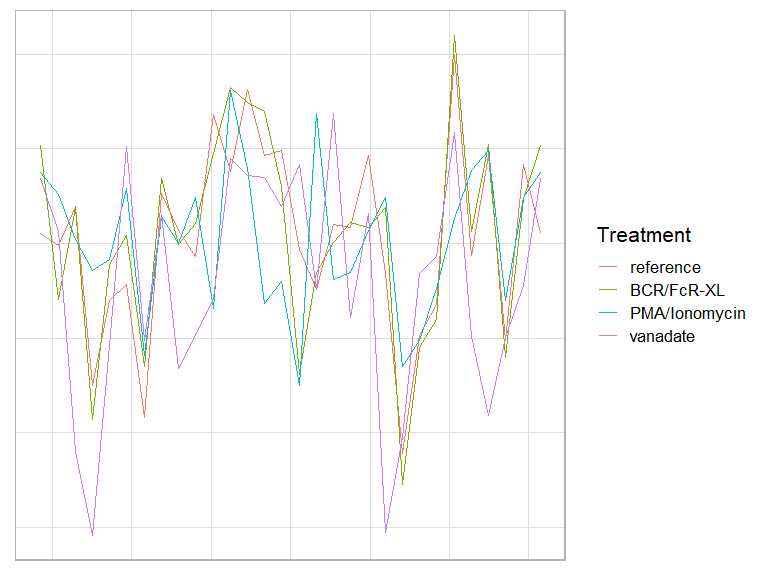Specific treatments are associated with changes in specific bins. Please note that this method compresses the Hilbert curve by only considering indices that contain cells. ## Projecting the Hilbert curve in 2 Dimensions The Hilbert curve can be re-folded back into any dimensionality using the hilbertProjection function. To visualize the Hilbert curve as a scatter plot, we use 2 as the target number of dimensions : proj <- hilbertProjection(hc,target = 2) ## Projecting the Hilbert curve to 2 dimensions will require 4096 bins To visualize the Hilbert curve as a 2D projection, we first compute the number of cells per unique combination of annotation columns and Hilbert index: fullProj <- fullAnnots %>% mutate(HilbertIndex=hc) %>% group_by_at(vars(one_of(colnames(fullAnnots),'HilbertIndex'))) %>% count() %>% bind_cols(as.data.frame(proj[.$HilbertIndex+1,]))
fullProjCount <- fullProj %>%
ungroup() %>%
count(HilbertIndex,Treatment) %>%
arrange(desc(n))
kable(head(fullProjCount))
HilbertIndex Treatment n
516096 reference 4
523264 reference 4
523903 BCR/FcR-XL 4
524287 reference 4
524287 BCR/FcR-XL 4

The percentage of cells per treatment is visualized using ggplot2 :

fullProj %>%
group_by(Treatment) %>%
mutate(PC=n/sum(n)) %>%
ggplot(aes(x=V1,y=V2))+
geom_tile(aes(fill=PC),
width=24,
height=24)+
facet_wrap(~Treatment)+
theme_light(base_size=16)+
theme(axis.title.x=element_blank(),
axis.text.x=element_blank(),
axis.ticks.x=element_blank(),
axis.title.y=element_blank(),
axis.text.y=element_blank(),
axis.ticks.y=element_blank())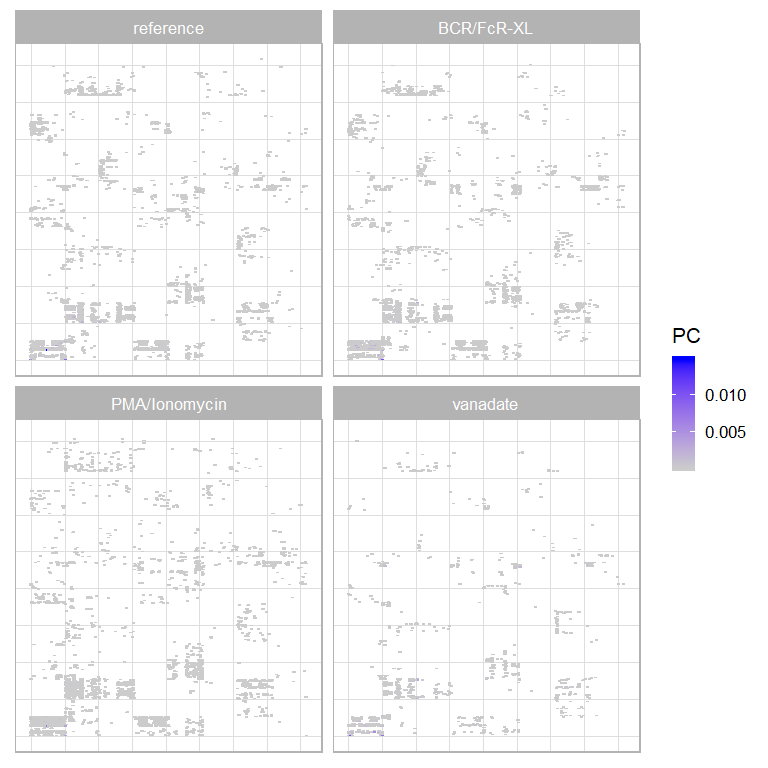The quality of the projection will depend on the density of the Hilbert curve. As this curve is sparse (only 0.16% of the Hilbert index contain at least 1 cell) this projection is only provided as an example.

# Comparing cells using Hilbert index

With each cell now associated to a specific hilbert index, each sample can be described by the percentage of cells from a given sample that corresponds to a particular index. The resulting table can be visualized as a heat map:

heatmap(log10(treatment.pc),
scale = 'none',
Colv = NA,
Rowv = NA,
labRow = NA,
col = colorRampPalette(c('white',blues9))(256),
margin = c(12,1))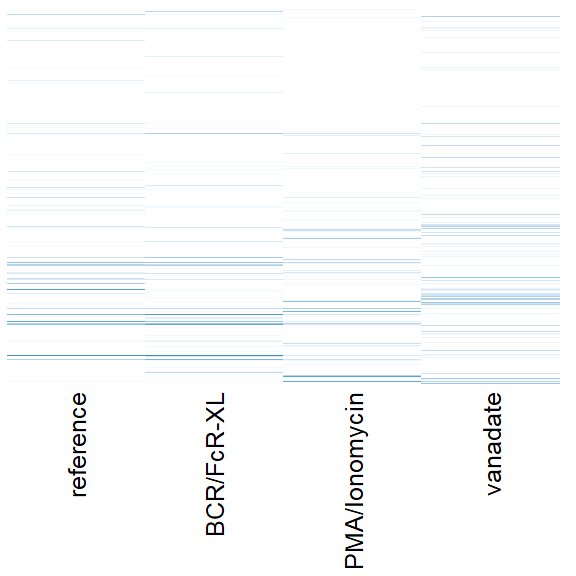In this experiment, each sample corresponds to a specific cell type and treatment: to compute the distance between samples we use a distance derived from information theory, the Jensen-Shannon distance. This is done through the js.dist function. The resulting distance matrix can be used to compute a hierarchical cluster:

treatment.dist <- js.dist(t(treatment.table))
treatment.hc <- hclust(treatment.dist)

When ordering samples using treatment.hc we see patterns emerging:

heatmap(log10(treatment.pc),
scale = 'none',
Colv = as.dendrogram(treatment.hc),
Rowv = NA,
labRow = NA,
col = colorRampPalette(c('white',blues9))(256),
margin = c(12,2))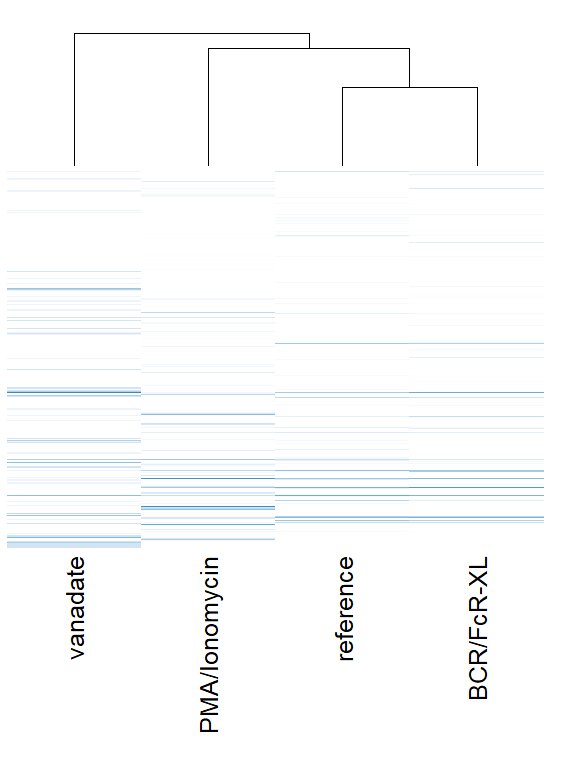Samples corresponding to reference and BCR/FcR-XL cluster together, while PMA/Ionomycin and vanadate samples show strong differences with every other sample.